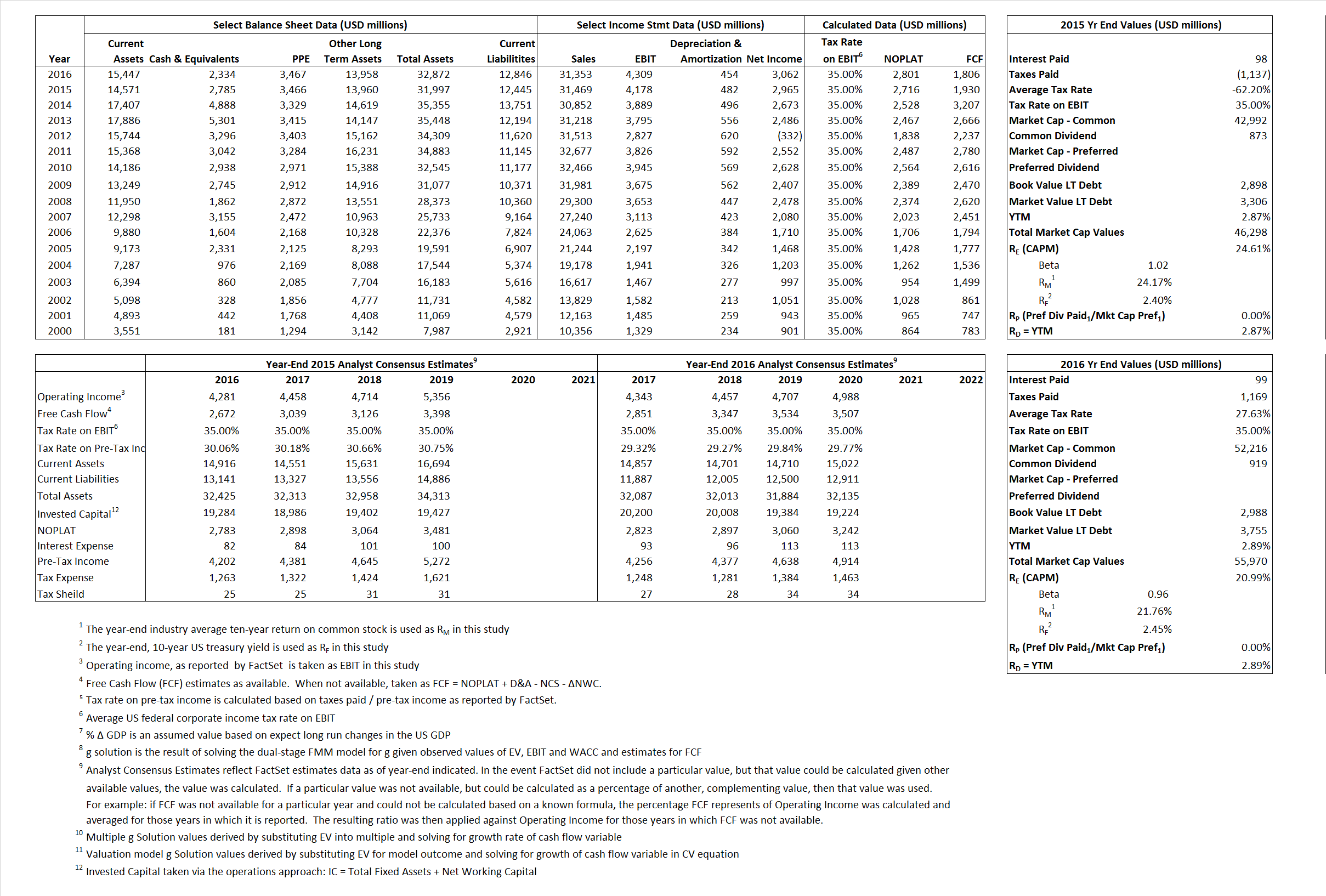# General Dynamics Corporation

## Analyst Listing

The following analysts provide coverage for the subject firm as of May 2016:

 Broker Analyst Analyst Email Deutsche Bank Research Myles Walton myles.walton@db.com Wolfe Research Hunter K. Keay hkeay@wolferesearch.com Credit Suisse Robert Spingarn robert.spingarn@credit-suisse.com Guggenheim Securities Roman Schweizer roman.schweizer@guggenheimpartners.com RBC Capital Markets Robert Stallard robert.stallard@rbccm.com CRT Capital Group Peter Arment peter.arment@bernstein.com Bernstein Research Douglas S. Harned douglas.harned@bernstein.com Cowen & Company Cai von Rumohr cai.von-rumohr@cowen.com Wells Fargo Securities Sam J. Pearlstein sam.pearlstein@wellsfargo.com Stifel Nicolaus Joseph W. DeNardi denardij@stifel.com Jefferies Howard A. Rubel hrubel@jefferies.com Buckingham Research Richard Safran rsafran@buckresearch.com

## Primary Input Data## Derived Input Data

### Equational Form

Net Operating Profit Less Adjusted Taxes NOPLAT  2,716  2,801$NOPLAT\, =\, EBIT\, x\, (1 \,-\, Avg \,\,Tax\,\, Rate\,\, on\,\, EBIT)$
Free Cash Flow FCF 1,930 1,806$FCF\,=NOPLAT\,+\,Non-Cash\,Expenses-\Delta NWC\,-\,NCS$
Tax Shield TS (61) 27$TS\,=\,Interest\,\,Paid\,\,x\,\, Avg \,\,Tax\,\,Rate\,\, on\,\, Pre-Tax\,\, Income$
Invested Capital IC  19,552 20,026$IC\,=\,Fixed\,\,Operating\,\,Assets\,\,+\,\,Net\,\, Working\,\, Capital$
Return on Invested Capital ROIC  13.89% 13.99%$ROIC\,=\,\frac { NOPLAT }{ IC }$
Net Investment NetInv  (1,570) 928$NetInv\,=\,{ {IC}_{1}}-{{IC}_{0}}+Depreciation$
Investment Rate IR  -57.81% 33.13%$IR\,=\,\frac {NetInv}{NOPLAT}$
Weighted Average Cost of Capital
WACCMarket  23.18%  19.72%$WACC\,=\,\frac { E }{ V } { R }_{ E }\,+\,\frac { P }{ V } { R }_{ P }\,+\,\frac { D }{ V } { R }_{ D }\left( 1- Avg\,\, Tax\,\,Rate\,\,on\,\,Pre-Tax\,\,Income \right)$
WACCBook    9.01%  8.24%
Enterprise value
EVMarket  43,513  53,636$EV\,=\,Market\,\,Cap\,\,Equity\,+\,\,Long\,\,Term\,\,Debt\,-\,Cash$
EVBook   43,105  52,870
Long-Run Growth
g = IR x ROIC
-8.03%   4.63% Long-run growth rates of the income variable are used in the Continuing Value portion of the valuation models.
g = %$\Delta$ GDP    2.50%   2.50%
Margin from Operations M  13.28%  13.74%$M\,\,=\,\,\frac{EBIT}{SALES}$
Depreciation/Amortization Rate D   10.34%  9.53%$D\,\,=\,\,\frac{D+A}{EBITDA}$

## Valuation Multiple Outcomes

The outcomes presented in this study are the result of original input data, derived data, and synthesized inputs.

### model g solution

12/31/2015 12/31/2016 12/31/2015 12/31/2016 12/31/2015 12/31/2016

EV/SALES$\frac {EV}{Sales} \,= \,\frac{ROIC\, -\, g}{ROIC\,(WACC\,-\,g)}\,(1\,-\,T)\,(M)$

1.38  1.71  30.76%  23.14%  26.03%  20.99%

EV/EBITDA$\frac {EV}{EBITDA} \,= \,\frac{ROIC\, -\, g}{ROIC\,(WACC\,-\,g)}\,(1\,-\,T)\,(1\,-\,D)$

9.34 11.26 30.76% 23.14% 26.03% 20.99%

EV/NOPLAT$\frac {EV}{NOPLAT} \,= \,\frac{ROIC\, -\, g}{ROIC\,(WACC\,-\,g)}$

16.02 19.15 30.76% 23.14% 26.03% 20.99%

EV/FCFOPS$\frac {EV}{FCF_{OPS}} \,= \,\frac{ROIC\, -\, g}{ROIC\,(WACC\,-\,g)}\,(1\,-\,T)$

22.55 29.70 30.76% 23.14% 26.03% 20.99%

EV/EBIT$\frac {EV}{EBIT} \,= \,\frac{ROIC\, -\, g}{ROIC\,(WACC\,-\,g)}\,(1\,-\,T)$

10.41 12.45 30.76% 23.14% 26.03% 20.99%

EV/IC$\frac {EV}{IC} \,= \,\frac{ROIC\, -\, g}{WACC\,-\,g}$

2.23  2.26 30.76% 23.14% 26.03% 20.99%